6.3 Discussion and Exercises

Binary trees have been used to model relationships for thousands of years. One reason for this is that binary trees naturally model (pedigree) family trees. These are the family trees in which the root is a person, the left and right children are the person's parents, and so on, recursively. In more recent centuries binary trees have also been used to model species trees in biology, where the leaves of the tree represent extant species and the internal nodes of the tree represent speciation events in which two populations of a single species evolve into two separate species.

Binary search trees appear to have been discovered independently by several groups in the 1950s [48, Section 6.2.2]. Further references to specific kinds of binary search trees are provided in subsequent chapters.

When implementing a binary tree from scratch, there are several design decisions to be made. One of these is the question of whether or not each node stores a pointer to its parent. If most of the operations simply follow a root-to-leaf path, then parent pointers are unnecessary, waste space, and are a potential source of coding errors. On the other hand, the lack of parent pointers means that tree traversals must be done recursively or with the use of an explicit stack. Some other methods (like inserting or deleting into some kinds of balanced binary search trees) are also complicated by the lack of parent pointers.

Another design decision is concerned with how to store the parent, left child, and right child pointers at a node. In the implementation given here, these pointers are stored as separate variables. Another option is to store them in an array,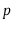, of length 3, so thatis the left child of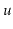,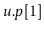is the right child of, and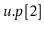is the parent of. Using an array this way means that some sequences of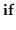statements can be simplified into algebraic expressions.

An example of such a simplification occurs during tree traversal. If a traversal arrives at a nodefrom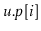, then the next node in the traversal is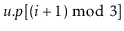. Similar examples occur when there is left-right symmetry. For example, the sibling ofis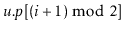. This trick works whetheris a left child (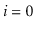) or a right child (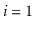) of. In some cases this means that some complicated code that would otherwise need to have both a left version and right version can be written only once. See the methodsand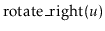on pagefor an example.

Exercise 6..1   Prove that a binary tree having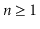nodes has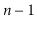edges.

Exercise 6..2   Prove that a binary tree havingreal nodes has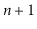external nodes.

Exercise 6..3   Prove that, if a binary tree,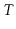, has at least one leaf, then either (a)'s root has at most one child or (b)has more than one leaf.

Exercise 6..4   Implement a non-recursive method,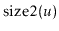, that computes the size of the subtree rooted at node.

Exercise 6..5   Write a non-recursive method,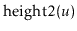, that computes the height of nodein a BinaryTree.

Exercise 6..6   A binary tree is size-balanced if, for every node, the size of the subtrees rooted at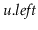and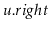differ by at most one. Write a recursive method,, that tests if a binary tree is balanced. Your method should run in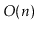time. (Be sure to test your code on some large trees with different shapes; it is easy to write a method that takes much longer thantime.)

A pre-order traversal of a binary tree is a traversal that visits each node,, before any of its children. An in-order traversal visitsafter visiting all the nodes in's left subtree but before visiting any of the nodes in's right subtree. A post-order traversal visitsonly after visiting all other nodes in's subtree. The pre/in/post-order numbering of a tree labels the nodes of a tree with the integers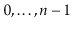in the order that they are encountered by a pre/in/post-order traversal. See Figure 6.10 for an example.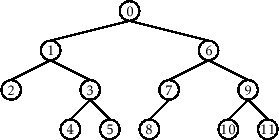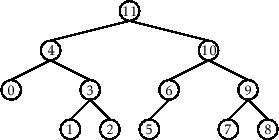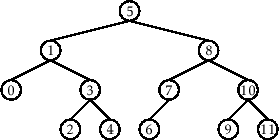Exercise 6..7   Create a subclass of BinaryTree whose nodes have fields for storing pre-order, post-order, and in-order numbers. Write recursive methods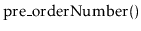,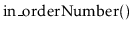, and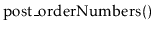that assign these numbers correctly. These methods should each run intime.

Exercise 6..8   Implement the non-recursive functions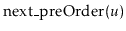,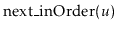, andthat return the node that followsin a pre-order, in-order, or post-order traversal, respectively. These functions should take amortized constant time; if we start at any nodeand repeatedly call one of these functions and assign the return value tountil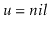, then the cost of all these calls should be.

Exercise 6..9   Suppose we are given a binary tree with pre-, post-, and in-order numbers assigned to the nodes. Show how these numbers can be used to answer each of the following questions in constant time:
1. Given a node, determine the size of the subtree rooted at.
2. Given a node, determine the depth of.
3. Given two nodesand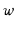, determine ifis an ancestor ofExercise 6..10   Suppose you are given a list of nodes with pre-order and in-order numbers assigned. Prove that there is at most one possible tree with this pre-order/in-order numbering and show how to construct it.

Exercise 6..11   Show that the shape of any binary tree on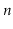nodes can be represented using at most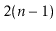bits. (Hint: think about recording what happens during a traversal and then playing back that recording to reconstruct the tree.)

Exercise 6..12   Illustrate what happens when we add the valuesand then 4.5 to the binary search tree in Figure 6.5.

Exercise 6..13   Illustrate what happens when we remove the values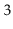and then 5 from the binary search tree in Figure 6.5.

Exercise 6..14   Implement a BinarySearchTree method,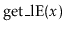, that returns a list of all items in the tree that are less than or equal to. The running time of your method should be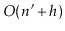whereis the number of items less than or equal to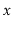and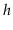is the height of the tree.

Exercise 6..15   Describe how to add the elementsto an initially empty BinarySearchTree in such a way that the resulting tree has height. How many ways are there to do this?

Exercise 6..16   If we have some BinarySearchTree and perform the operations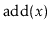followed by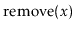(with the same value of) do we necessarily return to the original tree?

Exercise 6..17   Can aoperation increase the height of any node in a BinarySearchTree? If so, by how much?

Exercise 6..18   Can anoperation increase the height of any node in a BinarySearchTree? Can it increase the height of the tree? If so, by how much?

Exercise 6..19   Design and implement a version of BinarySearchTree in which each node,, maintains values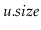(the size of the subtree rooted at),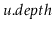(the depth of), and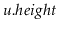(the height of the subtree rooted at).

These values should be maintained, even during calls to theandoperations, but this should not increase the cost of these operations by more than a constant factor.

opendatastructures.org